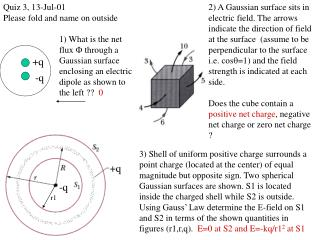DownloadDownload Presentation1) What is the net flux  through a Gaussian surface enclosing an electric dipole as shown to the left ?? 0

# 1) What is the net flux  through a Gaussian surface enclosing an electric dipole as shown to the left ?? 0

Télécharger la présentation## 1) What is the net flux  through a Gaussian surface enclosing an electric dipole as shown to the left ?? 0

- - - - - - - - - - - - - - - - - - - - - - - - - - - E N D - - - - - - - - - - - - - - - - - - - - - - - - - - -
##### Presentation Transcript

1. Quiz 3, 13-Jul-01 Please fold and name on outside 2) A Gaussian surface sits in electric field. The arrows indicate the direction of field at the surface (assume to be perpendicular to the surface i.e. cos=1) and the field strength is indicated at each side. Does the cube contain a positive net charge, negative net charge or zero net charge ? 1) What is the net flux  through a Gaussian surface enclosing an electric dipole as shown to the left ?? 0 +q -q 3) Shell of uniform positive charge surrounds a point charge (located at the center) of equal magnitude but opposite sign. Two spherical Gaussian surfaces are shown. S1 is located inside the charged shell while S2 is outside. Using Gauss’ Law determine the E-field on S1 and S2 in terms of the shown quantities in figures (r1,r,q). E=0 at S2 and E=-kq/r12 at S1 +q -q r1